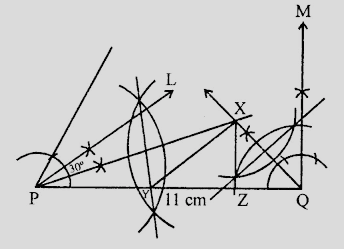# Construct a triangle $XYZ$ in which $\angle Y = 30^o , \angle Z = 90^o$ and $XY +YZ + ZX = 11\ cm$.

Given:

A triangle $XYZ$ in which $\angle Y = 30^o , \angle Z = 90^o$ and $XY +YZ + ZX = 11\ cm$.

To do:

We have to construct the given triangle.

Solution:Steps of construction:

(i) Draw a line segment $PQ =11\ cm$.

(ii) At $P$, draw a ray $PL$ making an angle of $30^o$ and $Q$, draw another ray $QM$ making an angle of $90^o$.

(iii) Draw the angle bisector of $\angle P$ and $\angle Q$ intersecting each other at $X$.

(iv) Draw the perpendicular bisectors of $XP$ and $XQ$ which intersect $PQ$ at $Y$ and $Z$ respectively.

(v) Join $XY$ and $XZ$.

Therefore,

$\triangle XYZ$ is the required triangle.

Updated on: 10-Oct-2022

19 Views